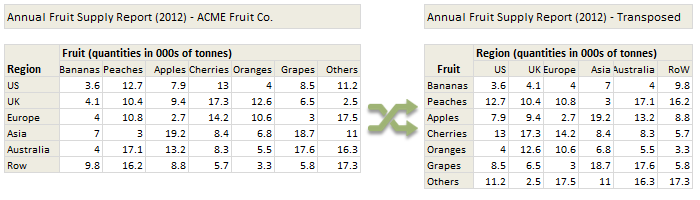# All articles with 'columns()' Tag

## Formula Forensics No. 34. Extract words from a cell, where they occur in a list of words.

Published on Sep 12, 2013 in Formula Forensics, Huis, Posts by HuiUse Excel to extract matching words from a list of words from a cell containing a sentence into individual cells in the order they exist

Continue »

## Transpose a table of data using Excel Formulas

Published on Feb 1, 2013 in Excel HowtosToday lets tackle a familiar data clean-up problem using Excel – Transposing data.

That is, we want to take all rows in our data & make them columns. Something like this:

Learn these 4 techniques to transpose data:
1. Using Paste Special > Transpose
2. Using INDEX formula & Helper cells
3. Using INDEX, ROWS & COLUMNS formulas
4. Using TRANSPOSE Formula

Continue »

## Automatic Rolling Months in Excel [Formulas]

Published on Apr 6, 2010 in Excel Howtos, Learn ExcelOften when we are making spreadsheets for forecasting or planning we would like to keep the starting month dynamic so that rest of the months in the plan can automatically rolled. Don’t understand? See this example: This type of setup is quite useful as it lets us change the starting month very easily. We can […]

Continue »

## Use ROWS() and COLUMNS() formulas to generate numbers in a sequence [quick tip]

Published on Aug 17, 2009 in Learn Excel

Here is a quick excel formula tip to start your week. Use ROWS() and COLUMNS() formulas next time you need sequential numbers. What does ROWS() excel formula do? ROWS excel formula takes a range as an argument and tells you how many rows are there in that range. For. eg. ROWS(A1:A10) gives 10. How can you […]

Continue »

### Get FREE Excel & Power-BI Newsletter

One email per week with Excel and Power BI goodness. Join 100,000+ others and get it free.